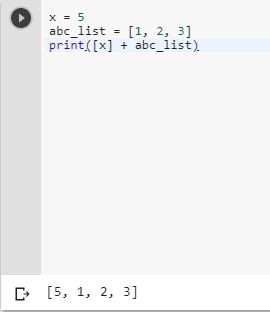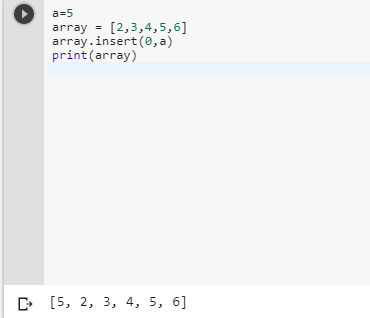2 views
in Python

I have an integer and a list. I would like to make a new list of them beginning with the variable and ending with the list. Writing a + list I get errors. The compiler handles as integer, thus I cannot use append, or extend either. How would you do this?

by (106k points)
• # For appending an integer to the beginning of the list in Python we can use the following method mentioned below.

• Now the reason why you were getting the error because you are using the variable name as the list that is not allowed in Python Python has some reserved keywords which can not be used as the variable name so instead of that, you should use the below-mentioned way:-

x = 5

abc_list = [1, 2, 3]

print([x] + abc_list)Another method that can be used to append an integer to the beginning of the list in Python is array.insert(index, value)this inserts an item at a given position. The first argument is the index of the element before which you are going to insert your element, so array.insert(0, x) inserts at the front of the list, and array.insert(len(array), x) is equivalent to array.append(x).

a=5

array = [2,3,4,5,6]

array.insert(0,a)

print(array)Wanna become an Expert in python? Come & join our Python Certification course